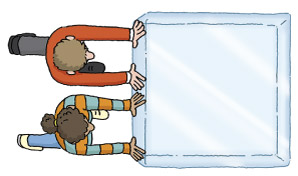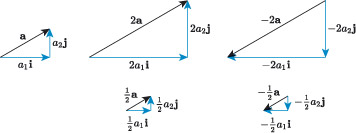Science, Maths & Technology

### Become an OU studentIntroducing vectors for engineering applications

Start this free course now. Just create an account and sign in. Enrol and complete the course for a free statement of participation or digital badge if available.

# 3.2 Scalar multiplication of vectors in component form

In Figure 27 Alice and Bob are both pushing the same face of the block of ice, but this time with the same force.Figure 27 Alice and Bob pushing a block of ice with the same force

Because they are both applying the same force, we can use a single vector to represent this, say , and if the force they apply is 110﻿ ﻿N, then

Now, the combined force exerted by both Alice and Bob is

This confirms that when we multiply a vector with a scalar quantity, the magnitude of the vector is multiplied by the scalar; if the scalar is positive its direction stays the same, but if the scalar is negative the direction is reversed.

For the situation of Alice and Bob pushing the block of ice we have

Other examples are illustrated in Figure 28. The vector can be written in component form as , and the scalar multiples of are written in component form as follows:

andFigure 28 Scalar multiplication of a vector , in component form

## Scalar multiplication of a vector in component form

If and is a scalar, then

In column notation, if and is a scalar, then

For example, if , then .

## Activity 15

Let and . Find each of the following scalar multiples.

• a.

• b.

• c.

• d.

• e.

• f.